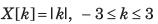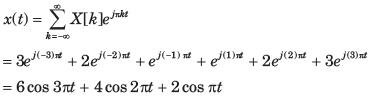Courses

# Test: Fourier Part - 1

## 10 Questions MCQ Test Signal and System | Test: Fourier Part - 1

Description
This mock test of Test: Fourier Part - 1 for Electrical Engineering (EE) helps you for every Electrical Engineering (EE) entrance exam. This contains 10 Multiple Choice Questions for Electrical Engineering (EE) Test: Fourier Part - 1 (mcq) to study with solutions a complete question bank. The solved questions answers in this Test: Fourier Part - 1 quiz give you a good mix of easy questions and tough questions. Electrical Engineering (EE) students definitely take this Test: Fourier Part - 1 exercise for a better result in the exam. You can find other Test: Fourier Part - 1 extra questions, long questions & short questions for Electrical Engineering (EE) on EduRev as well by searching above.
QUESTION: 1

### Determine the Fourier series coefficient for given periodic signal x(t). Q. x(t) as shown in fig. P5.7.1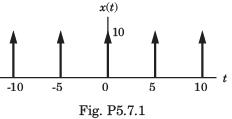Solution: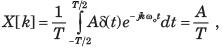A = 10 , T =  5, X [k] =  2

QUESTION: 2

### Determine the Fourier series coefficient for given periodic signal x(t). Q. x(t) as shown in fig. P5.7.2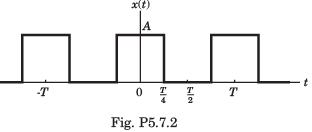Solution: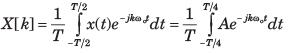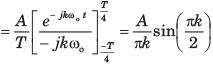QUESTION: 3

### Determine the Fourier series coefficient for given periodic signal x(t). Q. x(t) as shown in fig. P5.7.3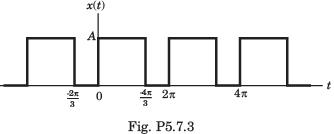Solution: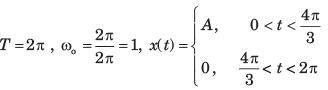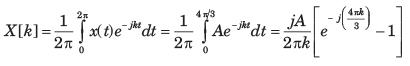QUESTION: 4

Determine the Fourier series coefficient for given periodic signal x(t).
Q. x(t) as shown in fig. P5.7.4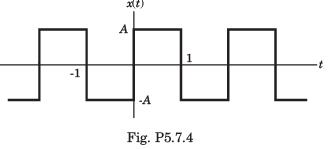Solution: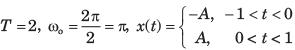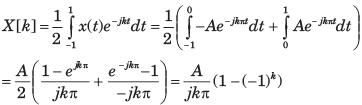QUESTION: 5

Determine the Fourier series coefficient for given periodic signal x(t).
Q. x(t) = sin2t

Solution: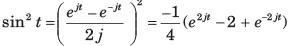The fundamental period of sin2( t) is π and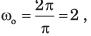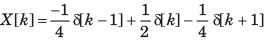QUESTION: 6

In the question, the FS coefficient of time-domain signal have been given. Determine the corresponding time domain signal and choose correct option.
Q.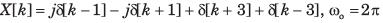Solution: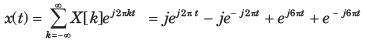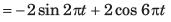QUESTION: 7

In the question, the FS coefficient of time-domain signal have been given. Determine the corresponding time domain signal and choose correct option.
Q.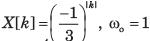Solution: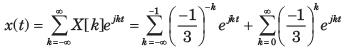QUESTION: 8

In the question, the FS coefficient of time-domain signal have been given. Determine the corresponding time domain signal and choose correct option.
Q. X [k] as shown in fig. P5.7.9,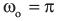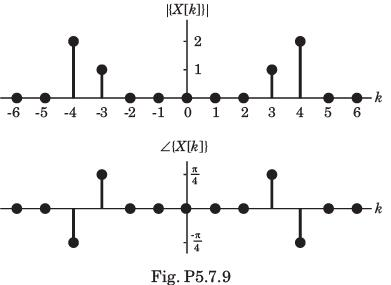Solution: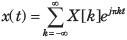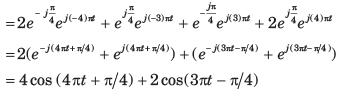QUESTION: 9

In the question, the FS coefficient of time-domain signal have been given. Determine the corresponding time domain signal and choose correct option.
Q. X [k] as shown in fig. P5.7.10,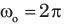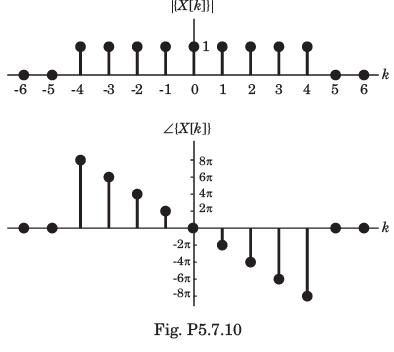Solution: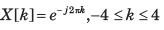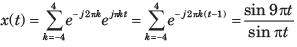QUESTION: 10

In the question, the FS coefficient of time-domain signal have been given. Determine the corresponding time domain signal and choose correct option.
Q. X [k] as shown in fig. P5.7.11,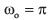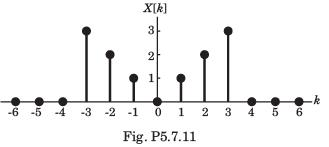Solution: